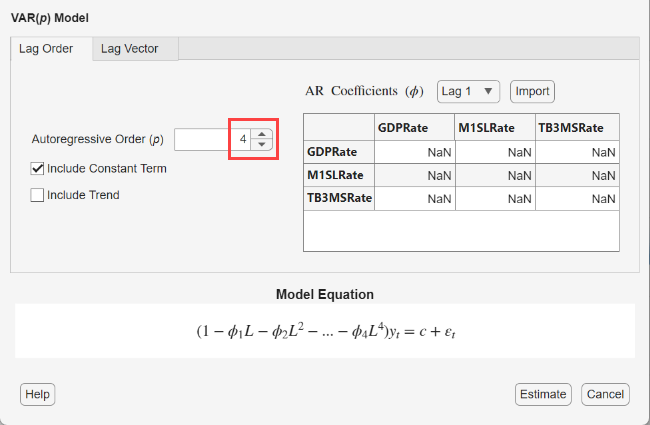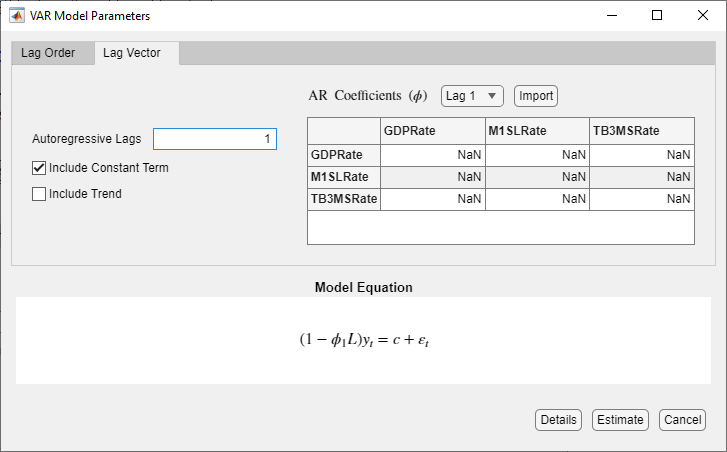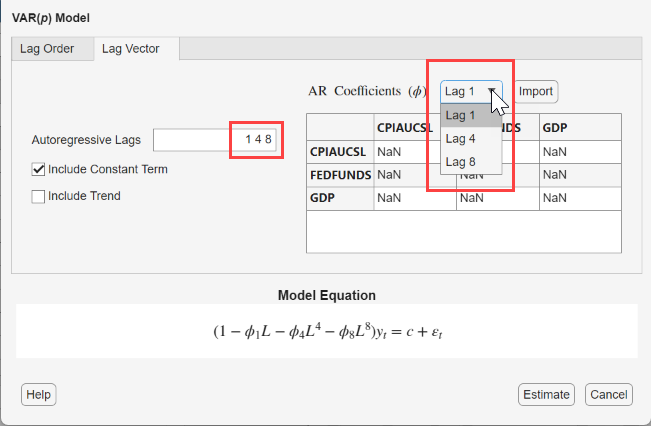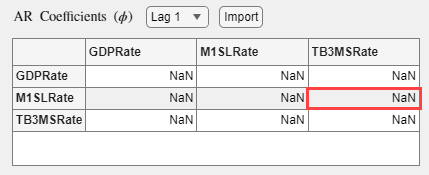## Specifying Multivariate Lag Operator Polynomials and Coefficient Constraints Interactively

Consider building a predictive, multivariate time series model (vector autoregression (VAR) or vector error-correction (VEC)) by using the Econometric Modeler app. After you choose candidate models for estimation (see Perform Exploratory Data Analysis), you can specify the model structure of each. To do so, on the Econometric Modeler tab, in the Time Series pane, select all series in the model, and then, in the Models section, click the model you want.After you select a time series model, the `Type` Model Parameters dialog box appears, where `Type` is the model type. For example, if you select VAR, then the VAR Model Parameters dialog box appears.• Econometric Modeler supports two options to specify the autoregressive (AR) or short-run lag operator polynomials. The adjustment options are on separate tabs: the Lag Order and Lag Vector tab. The Lag Order tab options offer a straight forward way to include consecutive lags from lag 1 (see Specify Lag Structure Using Lag Order Tab). The Lag Vector tab options allow you to create flexible models (see Specify Lag Structure Using Lag Vector Tab).

• Regardless of which lag adjustment option you choose, you can specify equality constraints for estimation on individual lag coefficients (entries) within the AR (for VAR models) or short-run (for VEC models) matrix. Similarly for VEC models, you can specify equality constraints on entire adjustment-speed and cointegration matrices. Econometric modeler enables you to enter equality constraints in the matrices provided in the dialog or import entire matrices containing constraints from the workspace. For more details, see Specify Coefficient Matrix Equality Constraints for Estimation.

• For verification, the model form appears in the Model Equation section. The model form updates to your specifications in real time.

### Specify Lag Structure Using Lag Order Tab

On the Lag Order tab, you can specify the orders of the lag operator polynomial by using the parameter appropriate for the model.

• For VAR or VARX models, use the Autoregressive Order box to specify the order of the autoregressive polynomial.

• For VEC models, use the Number of Lags box to specify the order of the short-run polynomial.

Type a nonnegative integer or click the appropriate arrow on. The app includes all consecutive lags from 1 through `L` in the polynomial, where `L` is the specified order.

When you specify an order, you can verify the lag operator polynomial in the Model Equation section. Also, Econometric Modeler includes all consecutive lags through `L` in the AR Coefficients (ϕ) (for VAR models) or Short-Run Coefficients (Φ) (for VEC models) list. The matrix below the list is the coefficient matrix of the selected lag, which you can use to specify equality constraints (see Specify Coefficient Matrix Equality Constraints for Estimation).

Consider a 3-D VAR(4) model. To specify this model using the parameters in the Lag Order tab:

1. Select the three time series of the model in the Time Series pane.

2. On the Econometric Modeler tab, in the Models section, click .

3. In the VAR Model Parameters dialog box, on the Lag Order tab, in the Autoregressive Order box, type `4`.4. Verify that the model in the Model Equation and the available lags in the AR Coefficients (ϕ) list contain lags 1 through 4.

### Specify Lag Structure Using Lag Vector Tab

On the Lag Vector tab, you specify a list of individual lags in the corresponding lag operator polynomial. This figure shows the Lag Vector tab in the VAR Model Parameters dialog box.To specify the lags that comprise each lag operator polynomial, type a list of nonnegative, unique integers in the corresponding box. Separate values by commas or spaces, or use the colon operator (for example, `4:4:12`).

Consider a VAR(8) model that includes only lags 1, 4, and 8. To specify this model using the parameters in the Lag Vector tab:

1. Select the three time series of the model in the Time Series pane.

2. On the Econometric Modeler tab, in the Models section, click .

3. In the VAR Model Parameters dialog box, on the Lag Vector tab, in the Autoregressive Lags box, type `1 4 8`.### Specify Coefficient Matrix Equality Constraints for Estimation

Econometric Modeler supports equality constraints when estimating the following parameters:

• For VAR and VARX models, you can specify equality constraints on individual entries of the AR coefficient matrices. In other words, you can specify known values for some matrix elements and estimate the others.

• For VEC models, you can specify equality constraints on:

• Individual entries of the short-run coefficient matrices, like the AR coefficients of VAR models.

• The entire adjustment-speed and cointegration matrices (that is, Econometric Modeler does not support constraints on individual matrix elements).

To specify equality constraints, enter the constraints in appropriate matrix provided in the `Type` Model Parameters dialog below the name of the matrix. By default, Econometric Modeler populates all matrices with `NaN` values, which indicate unknown, estimable parameters.

For all matrices, each row corresponds to a response equation in the model. For AR and short-run coefficient matrices, each column is the lag coefficient of the specified variable within the equation. For example, in the figure, entry (2,3) is the lag 1 coefficient of `TB3MSRate` in the equation of `M1SLRate`.For VEC models, adjustment-speed A and cointegration matrices B have the `r` linearly independent columns, where `r` is the rank of the cointegrating relation specified in the Rank box. Each column corresponds to a cointegrating relation.Econometric Modeler enables you to specify constraints in two ways:

• You can click on the elements you want to constrain in the matrices provided, and enter the constraint.

• You can use the button above the matrix to import an appropriately sized and configured matrix of constraints from the workspace.

For example, consider a VEC(1) model for short-, medium-, and long-term annual Canadian interest rate series from 1954 through 1994. Suppose economic theory suggests the following characteristics of the model:

• Each series is a unit root process with a cointegration rank of 2.

• The deterministic terms in the model are a vector of intercepts in the cointegrating relations and a deterministic linear trend vector in the levels of the data (H1 Johansen form).

• The linear effect, on the current long-run interest rate, of the short-run interest rate in the previous year is 0.5.

• The cointegrating relations that produce a stationary processes are 2.1`INT_L` - 2.1`INT_M` + 0.1`INT_S` and 1.7`INT_L` - 3.7`INT_M` + 1.8`INT_S`.

To specify this model and its characteristics, follow this procedure.

1. Load the Canadian inflation and interest rate data `Data_Canada.mat`.

`load Data_Canada`
2. At the command line, open the Econometric Modeler app.

`econometricModeler`

Alternatively, open the app from the apps gallery (see Econometric Modeler).

3. Select the series `INT_L`, `INT_M`, and `INT_S` in the Time Series pane.

4. On the Econometric Modeler tab, in the Models section, click .

5. In the VEC Model Parameters dialog, in the Rank box, type `2`.

6. In the matrix below the Short-Run Coefficients (Φ) list, in element (1,3), type `0.5`.

7. Below Cointegration Matrix (B), observe the order of the variables in the matrices is `INT_L`, `INT_M`, and `INT_S`. At the command line, create the cointegration matrix with the corresponding variable order

`B = [2.1 1.7; -2.1 -3.7; 0.1 1.7];`
In the VEC Model Parameters dialog, at Cointegration Matrix (B), click . In the dialog, select B.

The figure shows the parameter configurations in the VEC Model Parameters dialog.